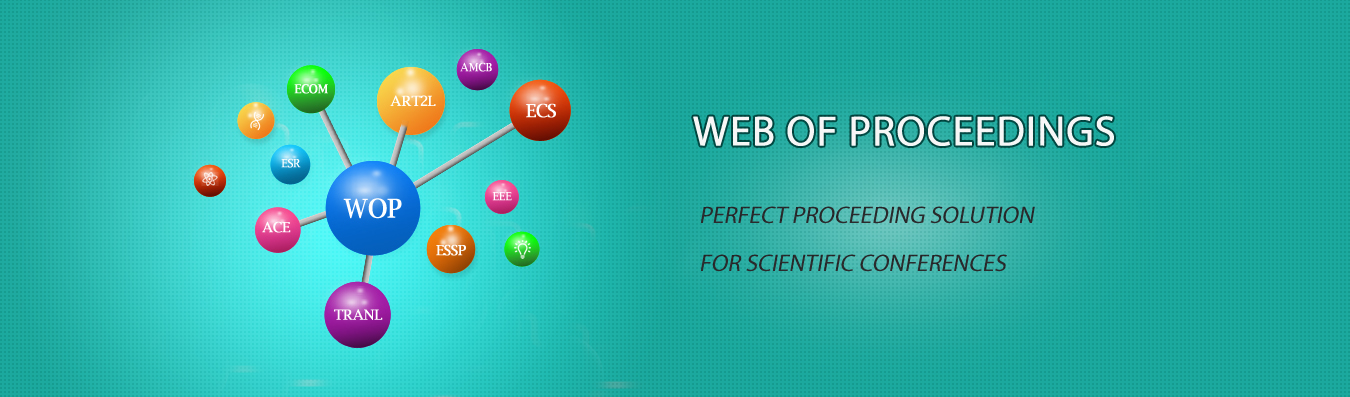The best way to conference proceedings by Francis Academic Press### Some Opinions on the Logic Structure of Mathematical Analysis

DOI: 10.25236/ietrc.2020.130

#### Author(s)

D. Luo, C. Wang, X. Tang

D. Luo

#### Abstract

The In this paper, the logic structure of the mathematical analysis and its reason are considered. At first, from the essence of the world in sociology, explain the reason that the objects of this subject are functions according to the equivalence between the functions and determinate relationships. Then explain that the suitable and basic tool for studying functions is limit for it is a bridge between the macrocosm and microcosm. Furthermore, according to the three fundamental characters of relationship: stability, variation, and accumulation during a continuous change process, analyze the stability through functions’ continuity with limit, describe the change of relationship via functions’ derivatives, and argue the total effect of continuous change by integral. At last, discuss the other parts of the structure of mathematical analysis and give their causes, which show that the structure of mathematical analysis is a complete subject system with strong logic.

#### Keywords

Logic structure, Mathematical analysis, Subject of mathematical, Research tools for mathematical analysis, The content of mathematical